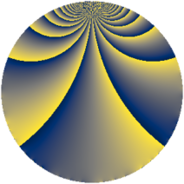# Properties

 Label 3744.1.ckLevel $3744$ Weight $1$ Character orbit 3744.ck Rep. character $\chi_{3744}(607,\cdot)$ Character field $\Q(\zeta_{6})$ Dimension $4$ Newform subspaces $1$ Sturm bound $672$ Trace bound $0$

# Related objects

## Defining parameters

 Level: $$N$$ $$=$$ $$3744 = 2^{5} \cdot 3^{2} \cdot 13$$ Weight: $$k$$ $$=$$ $$1$$ Character orbit: $$[\chi]$$ $$=$$ 3744.ck (of order $$6$$ and degree $$2$$) Character conductor: $$\operatorname{cond}(\chi)$$ $$=$$ $$468$$ Character field: $$\Q(\zeta_{6})$$ Newform subspaces: $$1$$ Sturm bound: $$672$$ Trace bound: $$0$$

## Dimensions

The following table gives the dimensions of various subspaces of $$M_{1}(3744, [\chi])$$.

Total New Old
Modular forms 52 4 48
Cusp forms 20 4 16
Eisenstein series 32 0 32

The following table gives the dimensions of subspaces with specified projective image type.

$$D_n$$ $$A_4$$ $$S_4$$ $$A_5$$
Dimension 0 4 0 0

## Trace form

 $$4q + 2q^{5} + 2q^{9} + O(q^{10})$$ $$4q + 2q^{5} + 2q^{9} + 2q^{13} - 2q^{17} + 4q^{29} - 2q^{33} - 2q^{37} - 2q^{45} - 2q^{49} - 2q^{57} + 4q^{65} + 8q^{73} - 2q^{81} - 4q^{85} - 2q^{89} + 4q^{93} + O(q^{100})$$

## Decomposition of $$S_{1}^{\mathrm{new}}(3744, [\chi])$$ into newform subspaces

Label Dim. $$A$$ Field Image CM RM Traces $q$-expansion
$$a_2$$ $$a_3$$ $$a_5$$ $$a_7$$
3744.1.ck.a $$4$$ $$1.868$$ $$\Q(\zeta_{12})$$ $$A_{4}$$ None None $$0$$ $$0$$ $$2$$ $$0$$ $$q+\zeta_{12}^{5}q^{3}-\zeta_{12}^{4}q^{5}-\zeta_{12}^{4}q^{9}+\cdots$$

## Decomposition of $$S_{1}^{\mathrm{old}}(3744, [\chi])$$ into lower level spaces

$$S_{1}^{\mathrm{old}}(3744, [\chi]) \cong$$ $$S_{1}^{\mathrm{new}}(468, [\chi])$$$$^{\oplus 4}$$